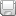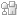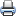Home > Articles > Cisco Network Technology > General Networking > Internet Addressing and Routing First Step

# Internet Addressing and Routing First StepSaveDiggDel.icio.usPrint

### Chapter Description

This chapter explains how internet routing works, and how internet addresses are assigned and utilized by network structures.

The Internet addressing protocol, or Internet Protocol (IP), is an additional addressing scheme. By following this protocol you can have an address with a structure that will allow you to build a scalable data communication system analogous to the postal system. Because this discussion is about computers, you need to decide how many bits you need for an Internet address, and how many Internet addresses you need. Computers can easily work with byte size pieces of data, so the number of bits in the IP should be a multiple of 8 bits or 1 byte. How many IP addresses will you need? That is, and was, a difficult question. When the IP was developed more than 20 years ago, the PC was not common, and it was difficult to imagine the explosion that would take place in the number of computers used throughout the world.

If you placed yourself back in 1980, and had to determine the size, in bits, of an IP address, what would you have picked? You might have started by determining how many addresses are possible based on the number of bytes that are used. And you might have created a table similar to Table 3-1.

#### Table 3-1 Number of IP Addresses Versus Number of Bytes

 Number of Bytes Number of Bits Number of Addresses 1 8 28 = 256 2 16 216 = 65,536 3 24 224 = 16,777,216 4 32 232 = 4,294,967,296 5 40 240 = 1,099,511,627,776 6 48 248 = 281,474,976,710,656

In 1980, there were more than 256 computers in use, so 1 byte would not be sufficient. Two bytes would give us 65,280 more addresses, but the number is still not sufficient. Although 3 bytes allow more than 16 million addresses, you know that computers are happier with even numbers of things than odd numbers of things. An ideal size is 4 bytes. Four is an even number and you can have in excess of 4 billion IP addresses, which should be sufficient. Now that you have settled on using a 32-bit address for the Internet address, you next need to determine a structure for those 32 bits.

The postal addressing and delivery schemes worked quite well for mail delivery, so let's try and impose the same type of structure on the Internet addresses. You know that a postal address has two components. The first component consisted of the state, city, and street names. The second component was the street or house number. Although the entire address was needed to identify a particular endpoint, or house, you did not need the street number for delivery until the mail reached the street containing the house. Using the same philosophy for the Internet address, use part of the 32 bits to designate the LAN or local network where the computer resides (the network address); and the remaining bits in the address to identify a particular computer, or host (the host address), on that LAN.

The next step is to determine how many bits to use for the network address and how many bits to use to identify a computer, or host, on that network. The easiest approach is to work with bytes, and then use the dotted decimal notation to represent network and host addresses using decimal numbers. You can't use all the bytes for the network address, and you can't use all the bytes for the computer address so the possibilities that remain are listed in Table 3-2.

#### Table 3-2 Internet Address Structures

 Network Address Size Number of Possible Networks Host Address Size Number of Possible Hosts 1 Byte 28 = 256 3 Bytes 224 = 16,777,216 2 Bytes 216 = 65,536 2 Bytes 216 = 65,536 3 Bytes 224 = 16,777,216 1 Byte 28 = 256

#### Table 3-3 Number of Hosts Possible

 Network Address Size Number of Possible Networks Host Address Size Number of Possible Hosts 1 Byte 28 = 256 3 Bytes 224 – 2 = 16,777,214 2 Bytes 216 = 65,536 2 Bytes 216 – 2 = 65,534 3 Bytes 224 = 16,777,216 1 Byte 28 – 2 = 254

Try working through the next exercises to reinforce your understanding.

For the first type or class of networks that use 1 byte for the network address and 3 bytes for the host address, the range of addresses in dotted decimal notation are:

```0.0.0.1–0.255.255.254 for network 0
1.0.0.1–1.255.255.254 for network 1
2.0.0.1–2.255.255.254 for network 2
...
255.0.0.1–255.255.255.254 for network 255```
1. What is the address for host 8 on network 129?

For the second class of networks that use 2 bytes for both the network and host addresses, the range of addresses in dotted decimal notation are

```0.0.0.1–0.0.255.254 for network 0
0.1.0.1–0.1.255.254 for network 1

...
255.254.0.1–255.254.255.254 for network 65,534
255.255.0.1–255.255.255.254 for network 65,535```
5. What is the address for host 8 on the 258th network?

6. Answer:@Answer. 1.1.0.8. The 258th network is network number 257 because network numbering started at 0; 257 in binary = 0000 0001 0000 0001 = 256 + 1 = 257 = 1.1 in dotted decimal notation.

For the third class of networks that use 1 byte for the host address and 3 bytes for the network address, the range of addresses in dotted decimal notation are

```0.0.0.1–0.0.0.254 for network 0
0.0.1.1–0.0.1.254 for network 1
...
0.1.0.1–0.1.0.254 for network 256
...
1.0.0.1–1.0.0.254 for network 65536
...
255.255.255.1–255.255.255.254 for network 16,777,215```
9. What is the address for host 8 on network 25?

11. What is the address for host 12 on network 103,481?

12. Answer:@103,481 in hexadecimal = 019439. 0116 = 110. 9416 = 14810. 3916 = 5710. 103,481 in dotted decimal = 1.148.57 so the address for host 12 on network 103,481 is 1.148.57.12

You must decide which scheme you are going to use for the Internet addresses. You could pick just one, but why not use all three? That way, you would have the flexibility of having networks with a few hosts (254), networks with a moderate number of hosts (65534), and networks with many hosts (16,777,214). This does sound like a good idea, but you need to be able to mix the three address types. A simple way is to use part of the first byte to signal the type of address used. The first byte, like all bytes, contains 8 bits. Using the first few bits to identify the type of network gives you the following rules:

• If the first, or most significant, bit of the first byte is 0, then 1 byte is used for the network address and 3 bytes are used for the host, broadcast, and "this" network address.

• If the most significant bit of the first byte is 1 and the next bit is 0, 2 bytes are used for the network address and 2 bytes are used for the host, broadcast, and "this" network address.

• If the first 2 bits of the first byte are 1, then 3 bytes are used for the network address and 1 byte is used for the host, broadcast, and "this" network address.

Three types of addresses are called Class A, B, and C. In a Class A address, the most significant bit of the first byte is 0. Additionally, you want to reserve two addresses from the Class A address space. Address 0.0.0.X (where X can be any value from 0–255) is reserved. Address 127.X.X.X is reserved for what is called a loopback address. The loopback address is used by a host to send a message to itself without even being connected to a network. This can be used for testing applications without interfering with the network. So the range of values for the first byte in a Class A address is 0000–0001–0111 1110 or 1–126 (0 and 127 are reserved).

The entire range of Class A addresses is – 126.255.255.255.

In a Class B address, the most significant bit of the first byte is 1 and the next bit is 0. So the range of values for the first byte in a Class B address is 1000 0000–1011 1111 or 128–191.

The entire range of Class B addresses is: 128.0.0.0–191.255.255.255.

In a Class C address, the first two most significant bits of the first byte are 1 and the next bit is 0. So the range of values for the first byte in a Class C address is 1100 0000–1101 1111 or 192–223.

The entire range of Class C addresses is: 192.0.0.0–223.255.255.255.

This Internet Protocol addressing scheme is called classful because every address falls into one of three classes of addresses as summarized in Table 3-4.

#### Table 3-4 Classful Internet Protocol Address Ranges

 Address Class First Host Address Last Host Address A 1.0.0.1 126.255.255.254 B 128.0.0.1 191.255.255.254 C 192.0.0.1 223.255.255.254

A Class A address is identified by the first bit being a 0, as follows:

`0XXX XXXX`

A Class B address is identified by the first 2 bits being 1 0, as follows:

`10XX XXXX`

And a Class C address has the first 3 bits set to 1 1 0, as follows:

`110X XXXX`

One question might have popped into your mind at this point: What about addresses that are not Class A, B, or C? In other words, what about addresses where the first four bits are 1 1 1 0 or 1 1 1 1? Addresses beginning with 1110 are a different class of addresses, Class D, which you will learn about in Chapter 9, "Multicast—What the Post Office Can't Do." The Class D, or multicast, address space is in the range 1110 0000–1110 1111 or 224–239.

Addresses beginning with 1111 are reserved for future use and cover the remaining address space starting at 240.0.0.0.

Figure 3-4 summarizes the structure of the classful IP addresses scheme.Figure 3-4 Classful IP Addressing Structures